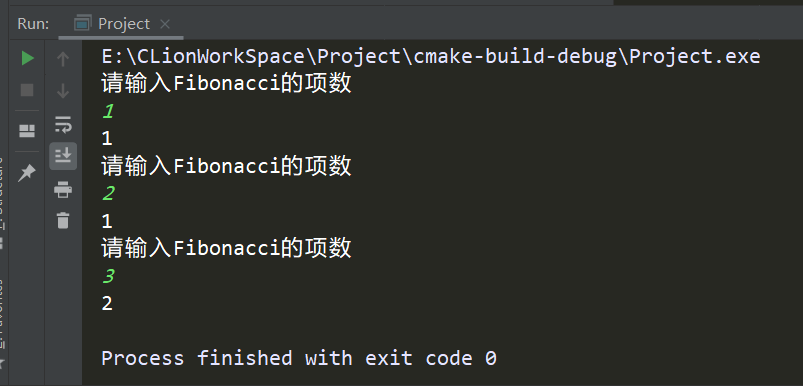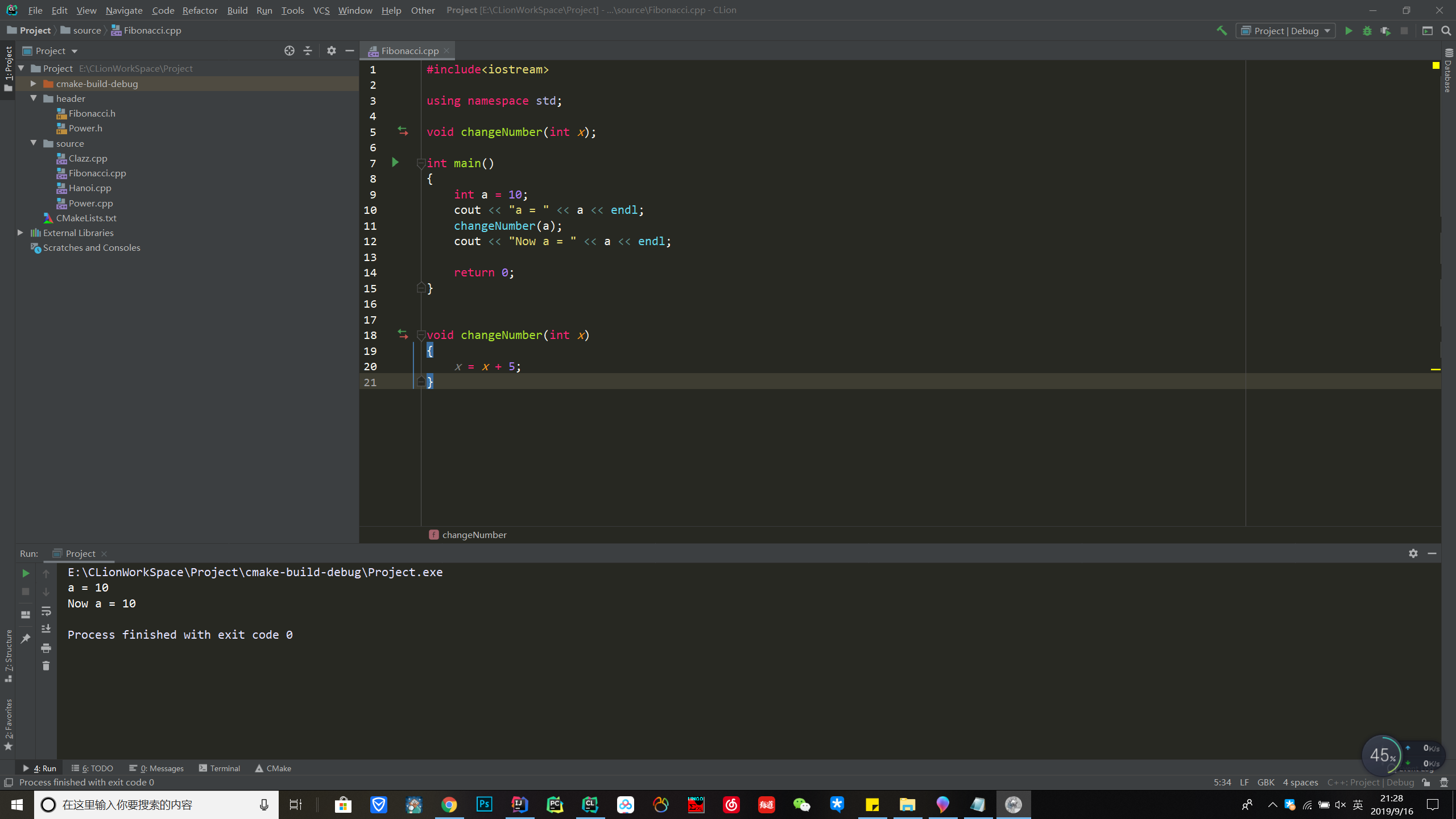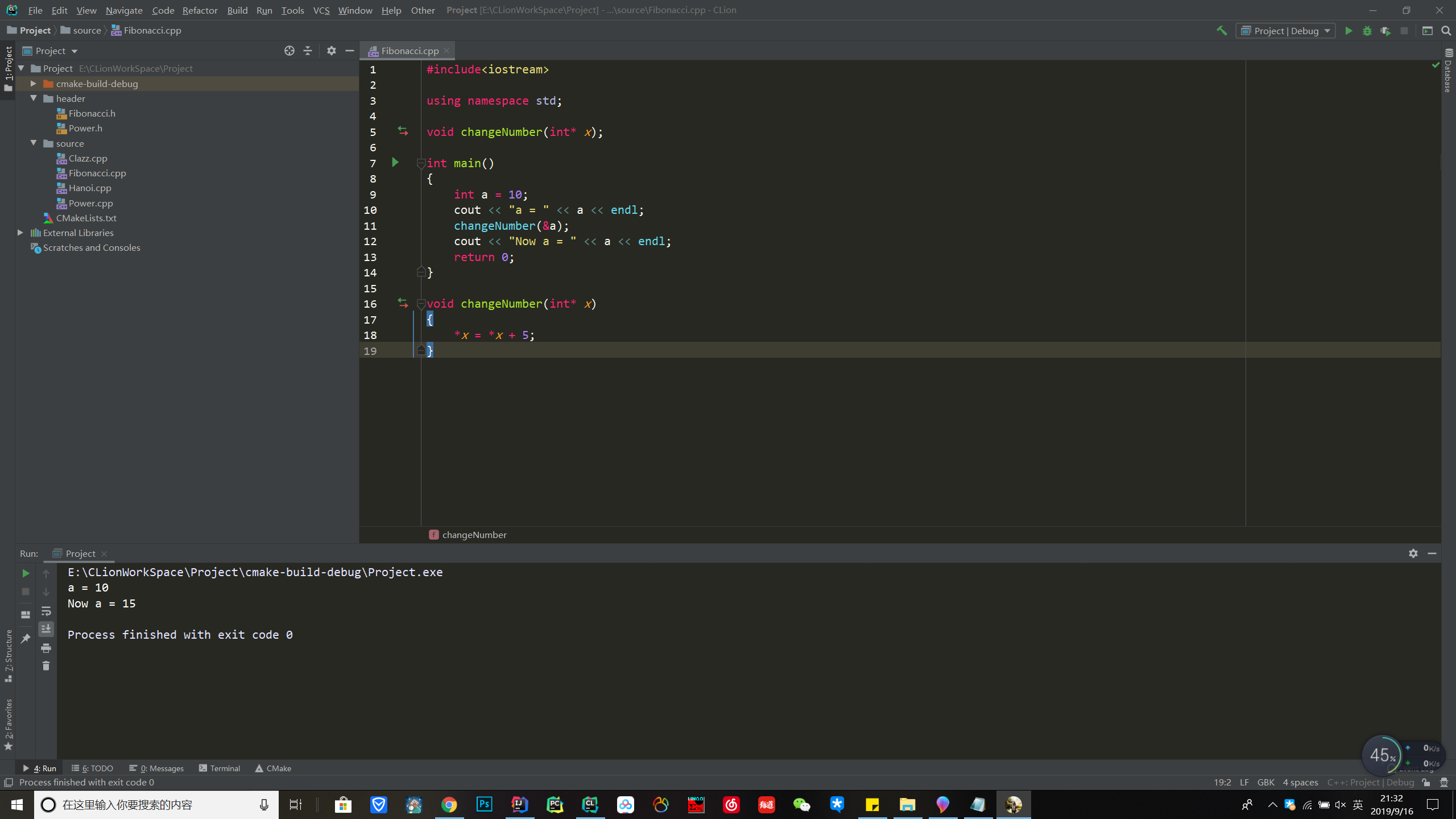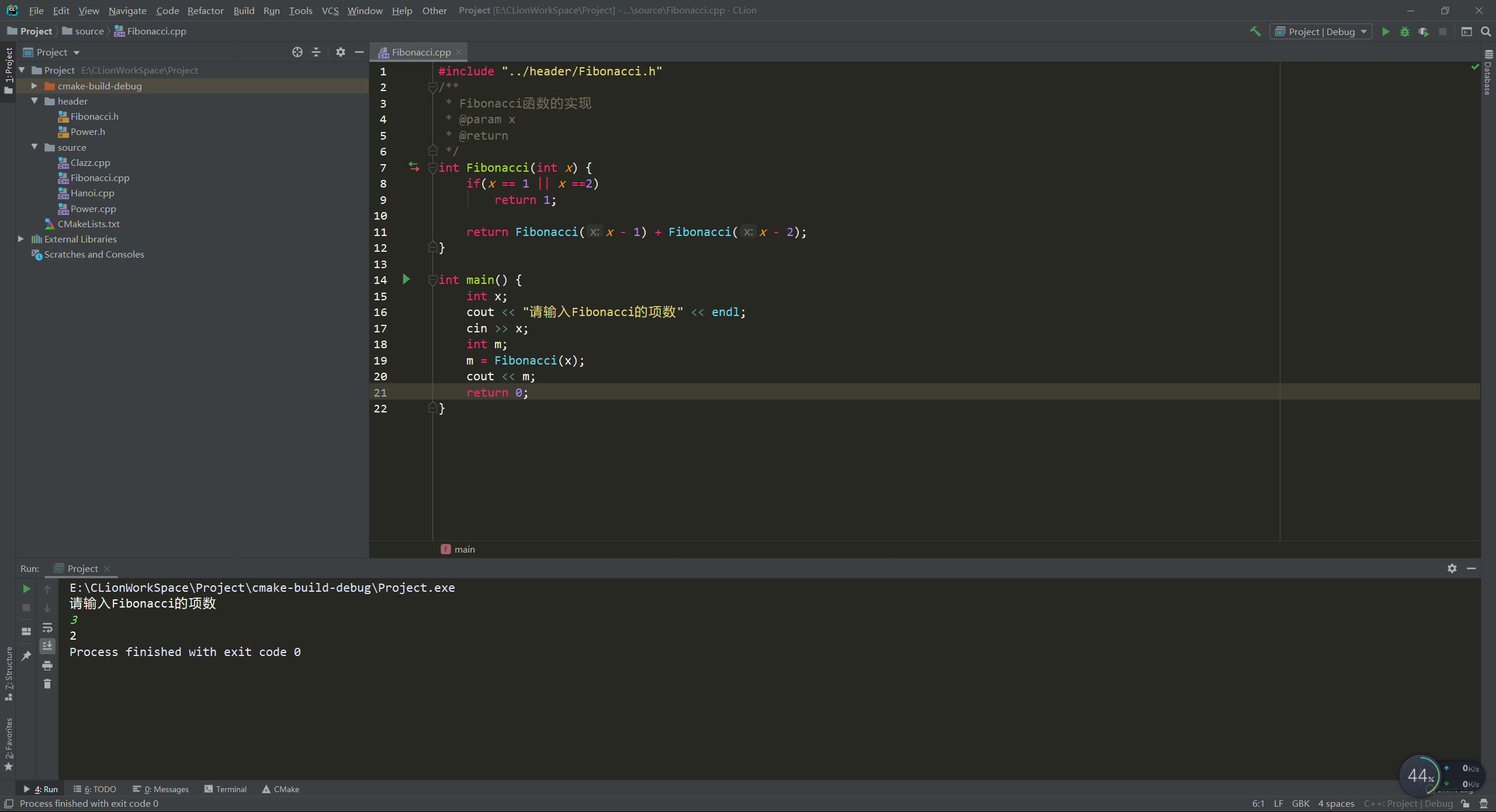# 1.为什么要用函数?

1. 实现代码的重用性
2. 易维护
3. 封装性（保密）
/**
* Fibonacci函数的实现
* @param x
* @return
*/
int Fibonacci(int x) {
if(x == 1 || x ==2)
return 1;

return Fibonacci(x - 1) + Fibonacci(x - 2);
}

int main() {
int x;
cout << "请输入Fibonacci的项数" << endl;
cin >> x;
int m;
m = Fibonacci(x);
cout << m;
return 0;
}# 2.为什么要用函数重载?

 void print_int(int a);
void print_char(char c);
void print_string(char *str);

 void print(int a);
void print(char c);
void print(char *str);

函数的名字仅仅是让编译器直到它调用的是哪个函数，用户并不关心函数的名字。而函数重载可以再一定程度上减轻程序员起名字，记名字的负担。

# 3.什么是值传递

#include<iostream>

using namespace std;

void changeNumber(int x);

int main()
{
int a = 10;
cout << "a = " << a << endl;
changeNumber(a);
cout << "Now a = " << a << endl;

return 0;
}

void changeNumber(int x)
{
x = x + 5;
}# 4.什么是地址传递？

#include<iostream>
using namespace std;
void changeNumber(int* x);
int main(void)
{
int a = 10;
cout << "a = " << a << endl;
changeNumber(&a);
cout << "Now a = " << a << endl;
return 0;
}
void changeNumber(int* x)
{
*x = *x + 5;
}

#这里的代码就是按地址传递，首先在changeNumber()的声明中，使用了int*类型而不是int类型，其次在main()函数调用changeNumber()函数的时候使用的实参是&a而不是a，这就传递了一个地址给changeNumber()函数，这个changeNumber()就可以完成修改a的任务。

# 5.如何编写递归函数？

递归，递归就是一个函数在它的函数体内调用它自身。执行递归函数将反复调用其自身，每调用一次就进入新的一层。

/**
* Fibonacci函数实现递归调用
* @param x
* @return
*/
int Fibonacci(int x) {
if(x == 1 || x ==2)
return 1;

return Fibonacci(x - 1) + Fibonacci(x - 2);
}

int main() {
int x;
cout << "请输入Fibonacci的项数" << endl;
cin >> x;
int m;
m = Fibonacci(x);
cout << m;
return 0;
}posted @ 2019-09-12 19:42  rnaNg0丶  阅读(283)  评论(0编辑  收藏  举报